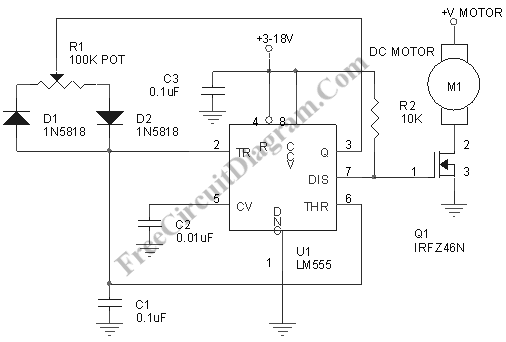# PWM Motor Control CircuitSpeed control for dc motor (electric motor) can be implemented using open loop or closed loop. Closed loop controller, also known as servo controller,  or a feedback control, gives the best performance since the loop will maintain the actual speed to follow the reference. In simple application, an open loop controller can be enough if the load is constant, such as in fan control (PC fan speed control). This dc motor control circuit uses PWM (pulse width modulation),  gives a better efficiency than using linear driver. Here is the schematic diagram of this PWM motor controller.The circuit uses the very popular 555 IC, but here the circuit is configured in unusual way. The PWM output is taken from the discharge pin, an open collector point that should be pulled high using a Vcc-connected resistor, while the original output pin (pin 3) is functioned to discharge the timing capacitor. The recommended supply voltage for 555 IC is between 9-12 volt, and you can connect the +V motor to this 555 supply voltage if the motor use 9-12 volt. The frequency of this PWM controller is about 144Hz, and you can change the frequency by changin the C1 capacitor, use smaller caps for higher frequency. The motor current can be as high as 37A if you allow the MOSFET to rise its case temperature to 100 C, and you must provide enough heat sink to maintain the temperature below this point. It is a good idea to provide protector diode in parallel with the motor to short the back-emf generated by the DC motor that might cause a voltage spike that could burn the MOSFET. This simple pwm control gives you a variable speed drives for motion control application. [Circuit schematic diagram source: dprg.org]